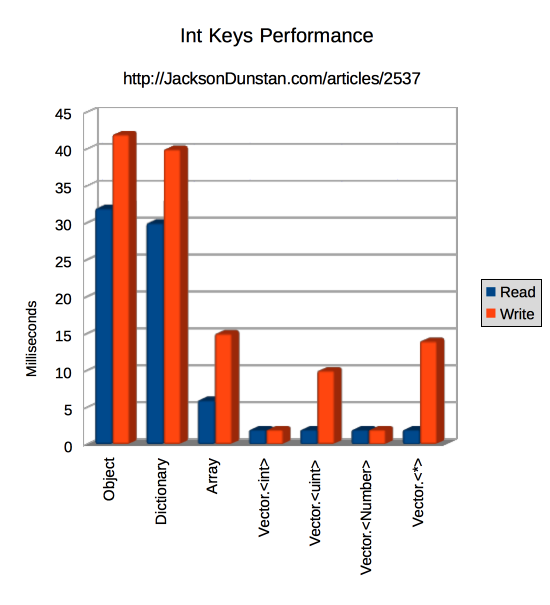Given that `Object` and `Dictionary` can have `int` keys and that `int` keys are faster than `String` keys, a natural performance test follows: which class is fastest at reading from and writing to those `int` keys? Is there a difference between the four `Vector` classes? Today’s article performs just that test and comes up with the answers.

The following test is very straightforward. It simply creates an `Object`, `Dictionary`, `Array`, and each of the four `Vector` classes (`int`, `uint`, `Number`, and `Boolean` for `*`), populates them with 1000 elements, and then reads and writes all of those elements 1000 times.

```package
{
import flash.display.*;
import flash.utils.*;
import flash.text.*;

public class IntKeysTest extends Sprite
{
private var logger:TextField = new TextField();
private function row(...cols): void
{
logger.appendText(cols.join(",") + "\n");
}

public function IntKeysTest()
{
stage.align = StageAlign.TOP_LEFT;
stage.scaleMode = StageScaleMode.NO_SCALE;

logger.autoSize = TextFieldAutoSize.LEFT;

init();
}

private function init(): void
{
const REPS:int = 1000;
const SIZE:int = 1000;
var i:int;
var j:int;
var valInt:int;
var valUint:uint;
var valNumber:Number;
var valBoolean:Boolean;
var beforeTime:int;
var afterTime:int;

var obj:Object = new Object();
var dict:Dictionary = new Dictionary();
var arr:Array = new Array();
var vecInt:Vector.<int> = new Vector.<int>();
var vecUint:Vector.<uint> = new Vector.<uint>();
var vecNumber:Vector.<Number> = new Vector.<Number>();
var vecObject:Vector.<Boolean> = new Vector.<Boolean>();
for (i = 0; i < SIZE; ++i)
{
obj[i] = i;
dict[i] = i;
arr[i] = i;
vecInt[i] = i;
vecUint[i] = i;
vecNumber[i] = i;
vecObject[i] = i;
}

row("Class", "Operation", "Time");

beforeTime = getTimer();
for (i = 0; i < REPS; ++i)
{
for (j = 0; j < SIZE; ++j)
{
valInt = obj[j];
}
}
afterTime = getTimer();

beforeTime = getTimer();
for (i = 0; i < REPS; ++i)
{
for (j = 0; j < SIZE; ++j)
{
valInt = dict[j];
}
}
afterTime = getTimer();

beforeTime = getTimer();
for (i = 0; i < REPS; ++i)
{
for (j = 0; j < SIZE; ++j)
{
valInt = arr[j];
}
}
afterTime = getTimer();

beforeTime = getTimer();
for (i = 0; i < REPS; ++i)
{
for (j = 0; j < SIZE; ++j)
{
valInt = vecInt[j];
}
}
afterTime = getTimer();

beforeTime = getTimer();
for (i = 0; i < REPS; ++i)
{
for (j = 0; j < SIZE; ++j)
{
valUint = vecUint[j];
}
}
afterTime = getTimer();

beforeTime = getTimer();
for (i = 0; i < REPS; ++i)
{
for (j = 0; j < SIZE; ++j)
{
valNumber = vecNumber[j];
}
}
afterTime = getTimer();

beforeTime = getTimer();
for (i = 0; i < REPS; ++i)
{
for (j = 0; j < SIZE; ++j)
{
valBoolean = vecObject[j];
}
}
afterTime = getTimer();

// Write

beforeTime = getTimer();
for (i = 0; i < REPS; ++i)
{
for (j = 0; j < SIZE; ++j)
{
obj[j] = valInt;
}
}
afterTime = getTimer();
row("Object", "Write", (afterTime-beforeTime));

beforeTime = getTimer();
for (i = 0; i < REPS; ++i)
{
for (j = 0; j < SIZE; ++j)
{
dict[j] = valInt;
}
}
afterTime = getTimer();
row("Dictionary", "Write", (afterTime-beforeTime));

beforeTime = getTimer();
for (i = 0; i < REPS; ++i)
{
for (j = 0; j < SIZE; ++j)
{
arr[j] = valInt;
}
}
afterTime = getTimer();
row("Array", "Write", (afterTime-beforeTime));

beforeTime = getTimer();
for (i = 0; i < REPS; ++i)
{
for (j = 0; j < SIZE; ++j)
{
vecInt[j] = valInt;
}
}
afterTime = getTimer();
row("Vector.<int>", "Write", (afterTime-beforeTime));

beforeTime = getTimer();
for (i = 0; i < REPS; ++i)
{
for (j = 0; j < SIZE; ++j)
{
vecUint[j] = valUint;
}
}
afterTime = getTimer();
row("Vector.<uint>", "Write", (afterTime-beforeTime));

beforeTime = getTimer();
for (i = 0; i < REPS; ++i)
{
for (j = 0; j < SIZE; ++j)
{
vecNumber[j] = valNumber;
}
}
afterTime = getTimer();
row("Vector.<Number>", "Write", (afterTime-beforeTime));

beforeTime = getTimer();
for (i = 0; i < REPS; ++i)
{
for (j = 0; j < SIZE; ++j)
{
vecObject[j] = valBoolean;
}
}
afterTime = getTimer();
row("Vector.<*>", "Write", (afterTime-beforeTime));
}
}
}```

Run the test

I ran this test in the following environment:

• Release version of Flash Player 12.0.0.70
• 2.3 Ghz Intel Core i7-3615QM
• Mac OS X 10.9.1
• ASC 2.0.0 build 354071 (`-debug=false -verbose-stacktraces=false -inline -optimize=true`)

And here are the results I got:

Class Operation Time
Object Write 42
Dictionary Write 40
Array Write 15
Vector.<int> Write 2
Vector.<uint> Write 10
Vector.<Number> Write 2
Vector.<*> Write 14`Object` and `Dictionary` are horribly slow compared to `Array` and the `Vector` classes at both reading and writing. They’re about 6x slower than the second-slowest `Array` at reading and 2-3x slower than the same at writing. In short, while they support `int` keys they should not be used for that purpose if you can get away with `Array` or a `Vector` class.

`Array`, in turn, is always slower than any `Vector` class. It’s 3x slower at reading, but writing is a more nuanced story. It’s only slightly slower than `Vector.<*>` and about ⅓ slower than `Vector.<uint>`. Compared to `Vector.<int>` and `Vector.<Number>`, however, it’s about 7x slower.

While all of the `Vector` classes have identical read performance, their write performances therefore vary. With a ~7x gap between the fast versions (`int` and `Number`) and the slow versions (`uint` and `*`), you’d be wise to pay attention to the particular type you’re storing in the `Vector` umbrella class. Still, if you can live with its restrictions you’ll always do best by using a `Vector` for your `int` keys mapping purposes.